This site uses cookies. By continuing to browse the ConceptDraw site you are agreeing to our Use of Site Cookies.
ConceptDraw Samples | Software Development — SYSML

# SYSML

The SysML diagrams are created using ConceptDraw DIAGRAM diagramming and vector drawing software enhanced with SysUML solution from ConceptDraw Solution Park.

ConceptDraw DIAGRAM provides export of vector graphic multipage documents into multiple file formats: vector graphics (SVG, EMF, EPS), bitmap graphics (PNG, JPEG, GIF, BMP, TIFF), web documents (HTML, PDF), PowerPoint presentations (PPT), Adobe Flash (SWF).

## Tutorials and Solutions:

Video Tutorials: ConceptDraw Solution Park

Solutions: SYSML for ConceptDraw DIAGRAM

## Sample 1: SysML Diagram — Builder Design Pattern Sequence

SYSML sample: Builder design pattern sequence.

This example is created using ConceptDraw DIAGRAM diagramming software enhanced with SYSML solution from ConceptDraw Solution Park.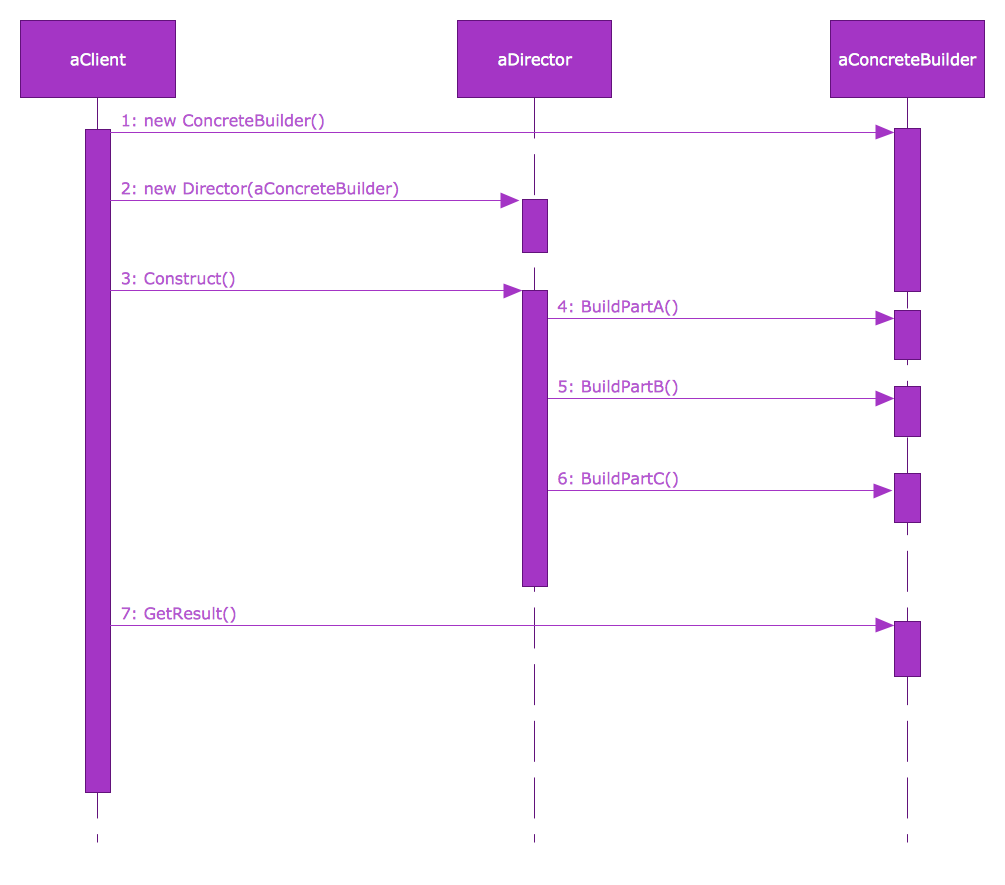## Sample 2: SysML Diagram — Identity Requirements Diagram

SYSML sample: Identity requirements diagram.

This example is created using ConceptDraw DIAGRAM diagramming software enhanced with SYSML solution from ConceptDraw Solution Park.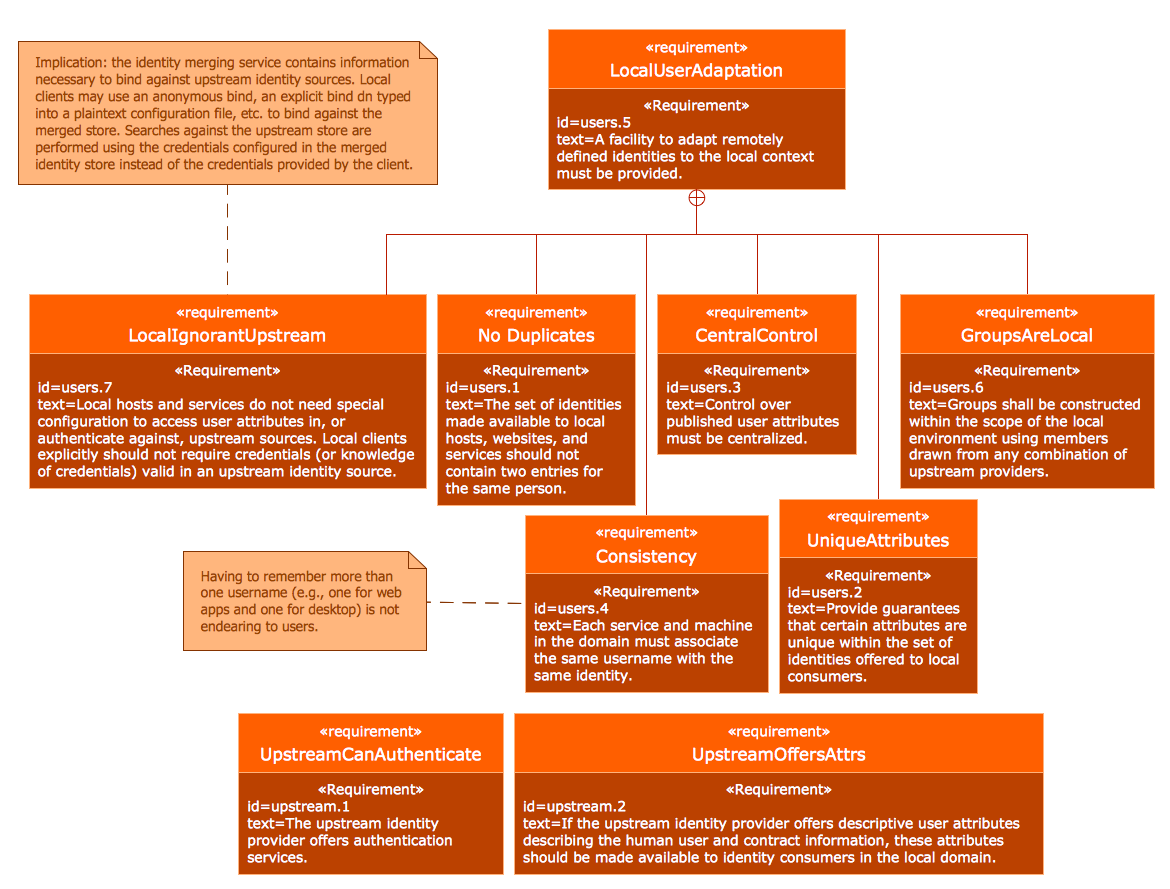## Sample 3: SysML Activity Diagram

SYSML sample: SysML activity diagram.

This example is created using ConceptDraw DIAGRAM diagramming software enhanced with SYSML solution from ConceptDraw Solution Park.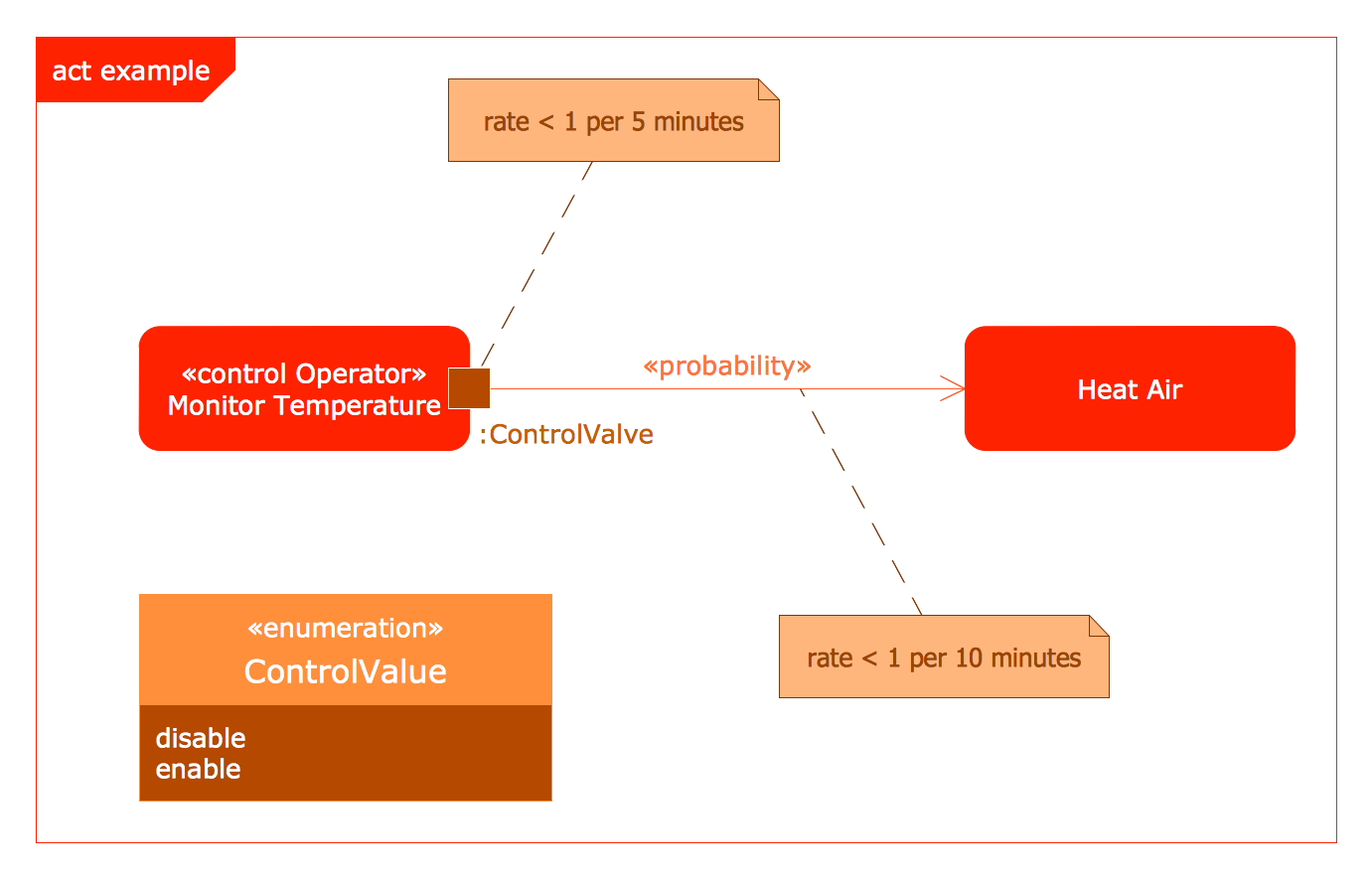## Sample 4: SysML Block Definition Diagram

SYSML sample: SysML block definition diagram.

This example is created using ConceptDraw DIAGRAM diagramming software enhanced with SYSML solution from ConceptDraw Solution Park.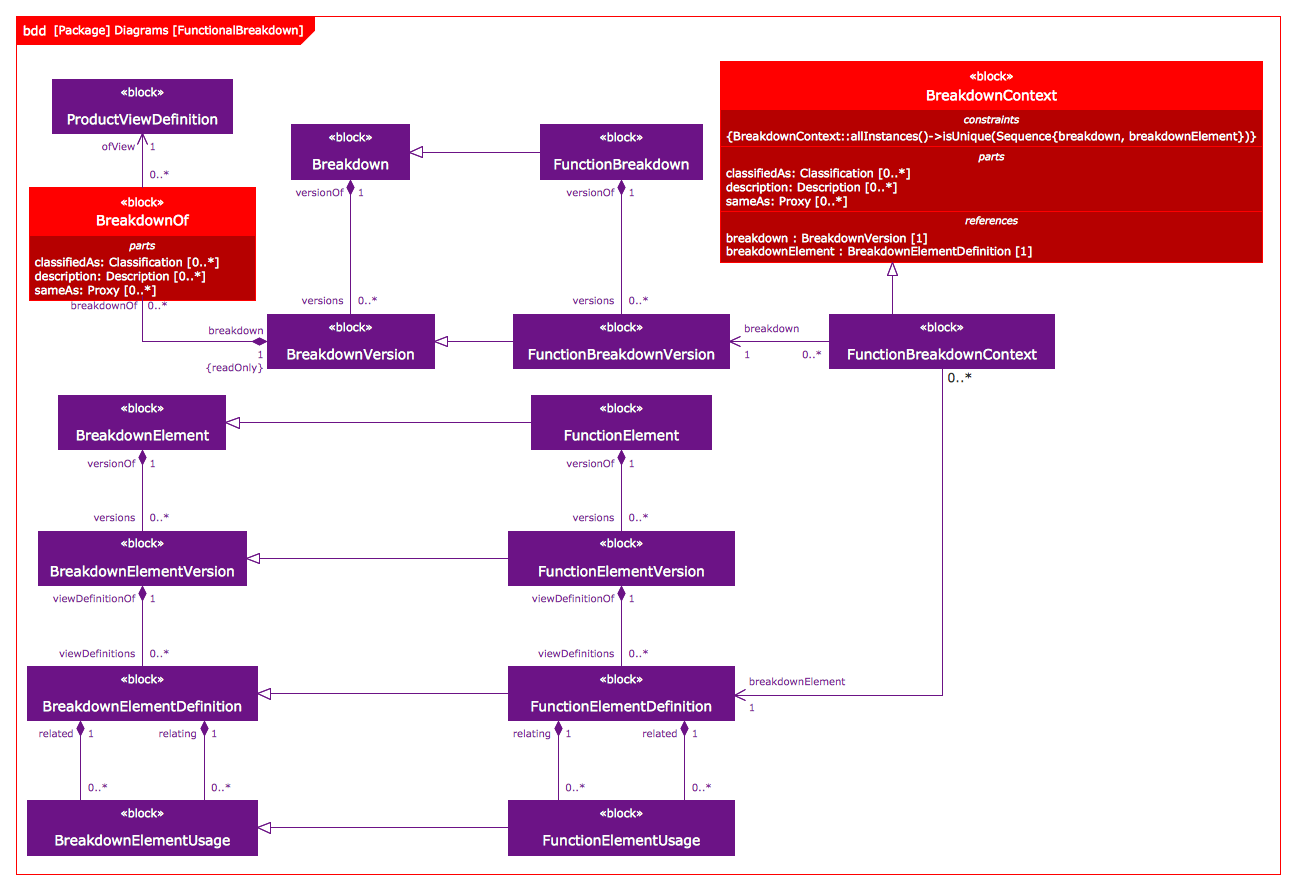## Sample 5: Use Case Restaurant Model

SYSML sample: Use case restaurant model.

This example is created using ConceptDraw DIAGRAM diagramming software enhanced with SYSML solution from ConceptDraw Solution Park.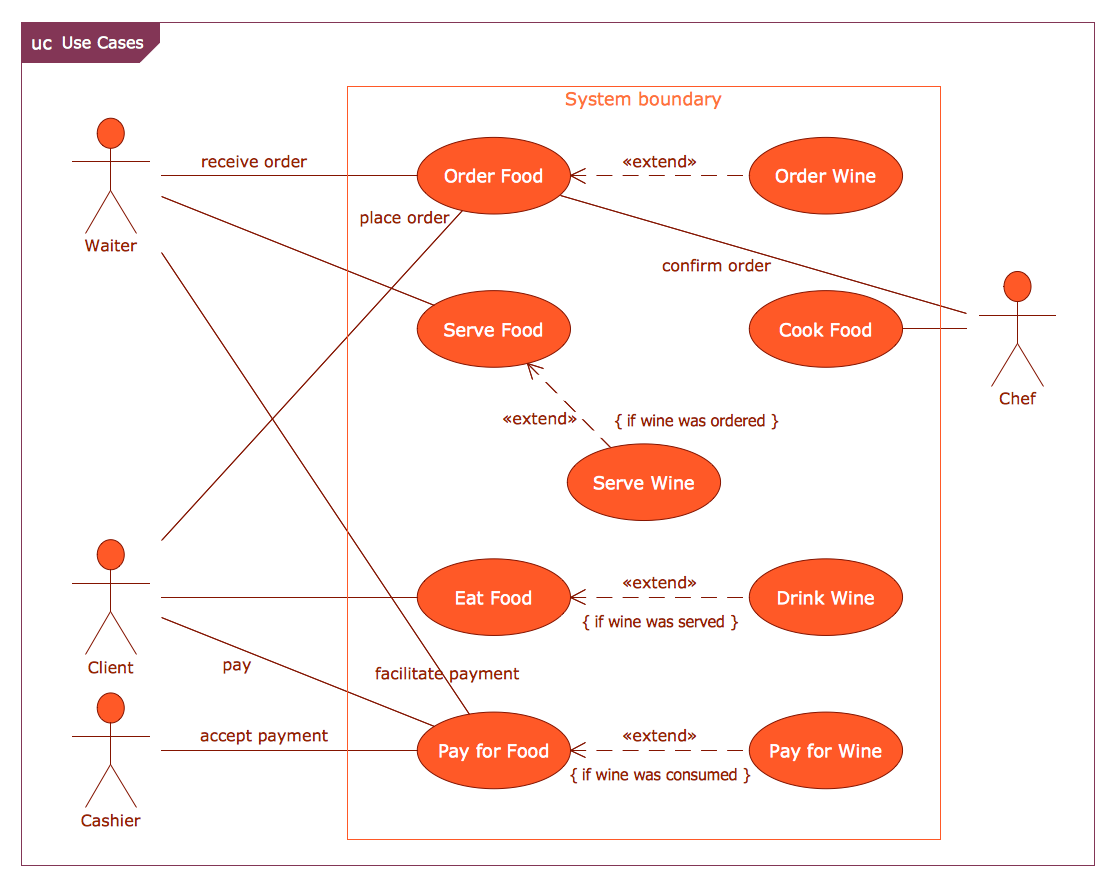## Sample 6: Package Diagram — Deployment Model Structure

SYSML sample: Package diagram — deployment model structure.

This example is created using ConceptDraw DIAGRAM diagramming software enhanced with SYSML solution from ConceptDraw Solution Park.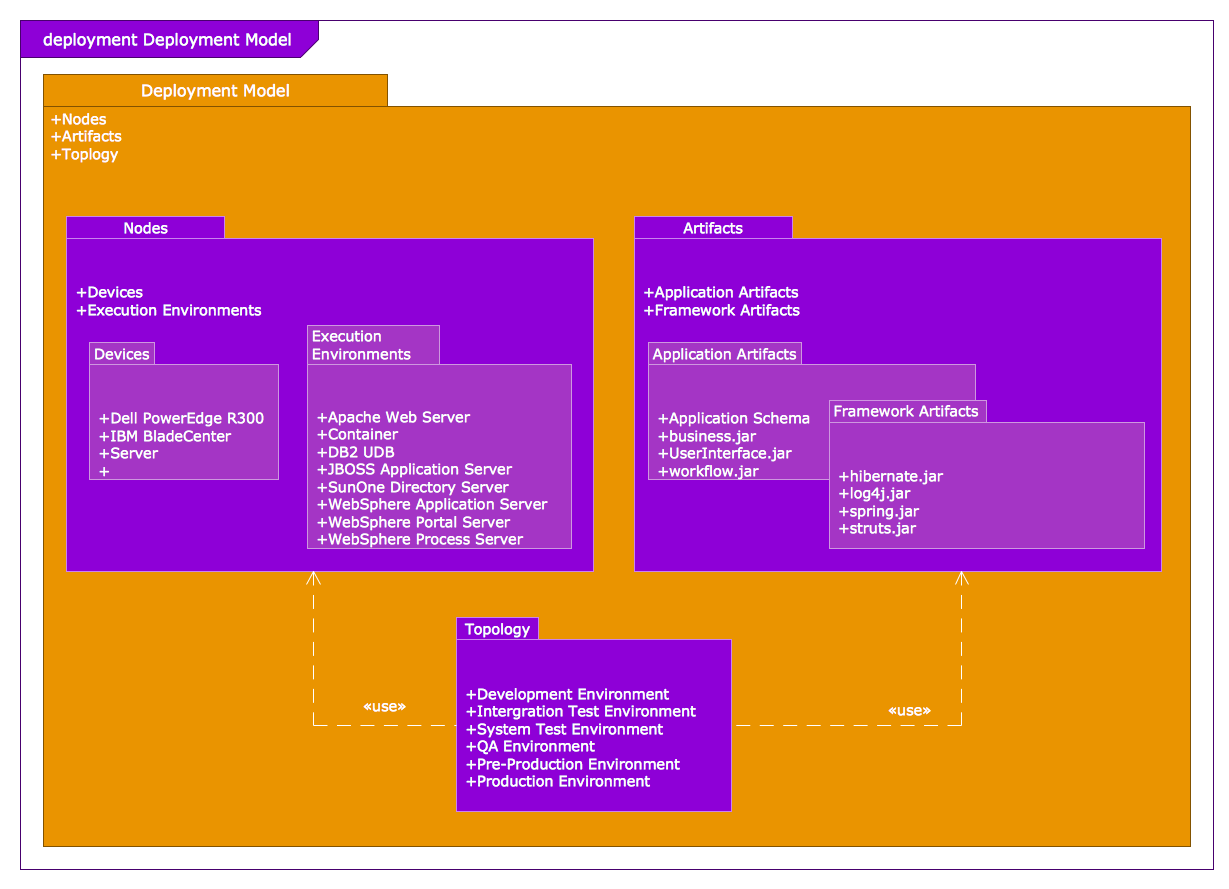## Sample 7: Parametric Diagram

SYSML sample: Parametric diagram.

This example is created using ConceptDraw DIAGRAM diagramming software enhanced with SYSML solution from ConceptDraw Solution Park.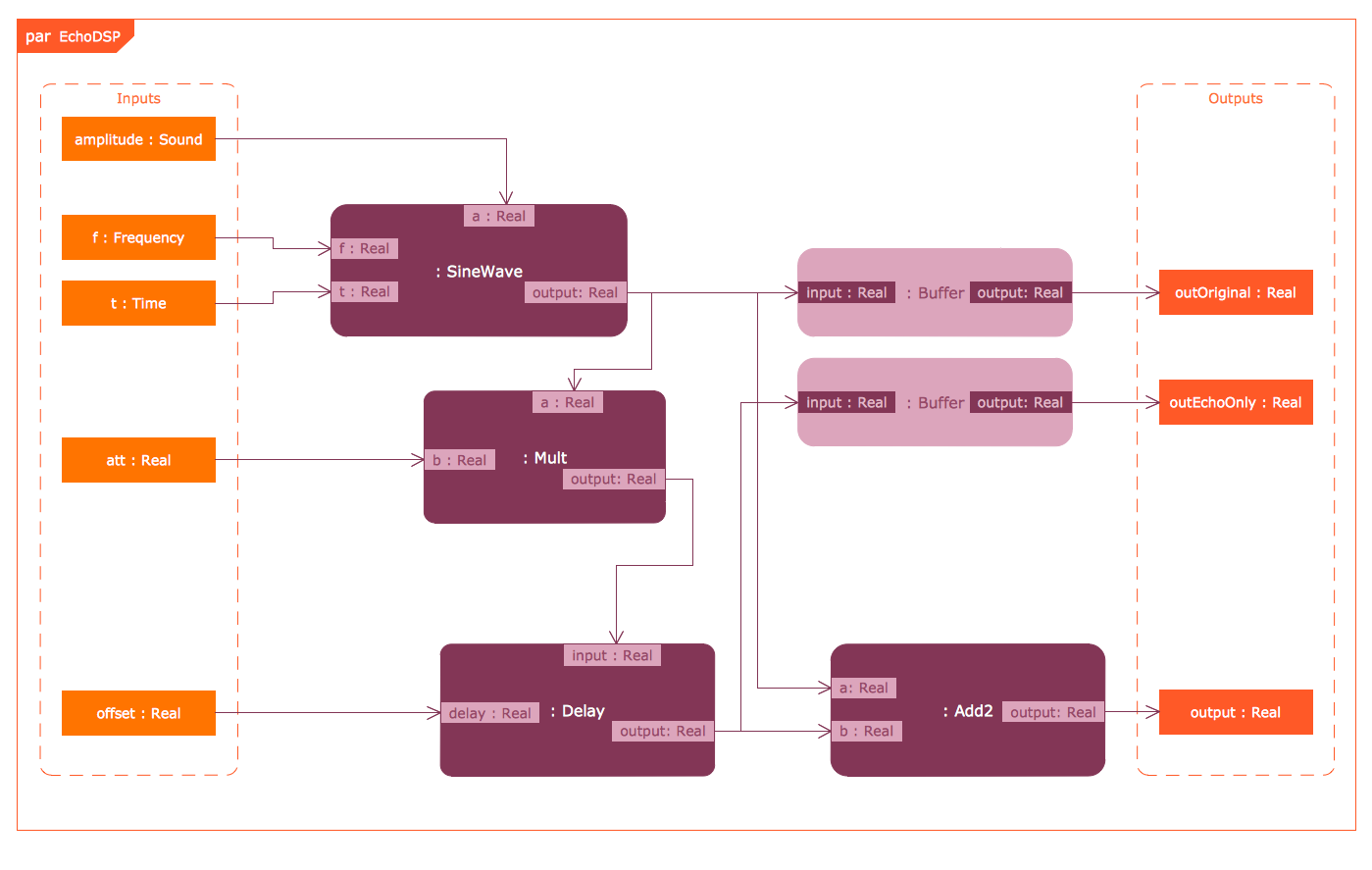## Sample 8: State Machine Diagram

SYSML sample: State machine diagram.

This example is created using ConceptDraw DIAGRAM diagramming software enhanced with SYSML solution from ConceptDraw Solution Park.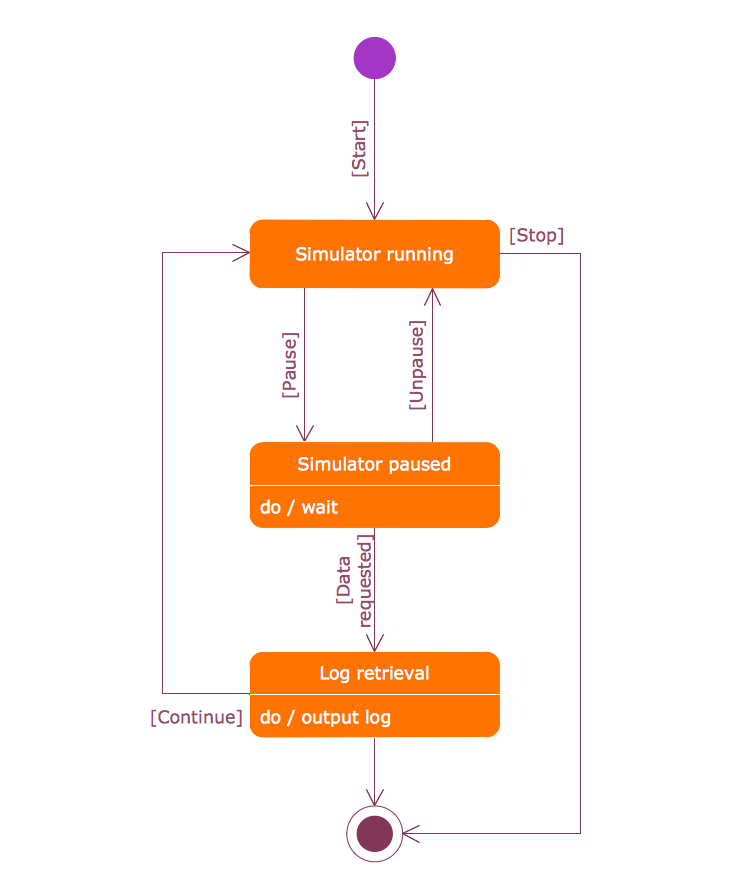All samples are copyrighted CS Odessa's.

Usage of them is covered by Creative Commons “Attribution Non-Commercial No Derivatives” License.

The text you can find at: https://creativecommons.org/licenses/by-nc-nd/3.0